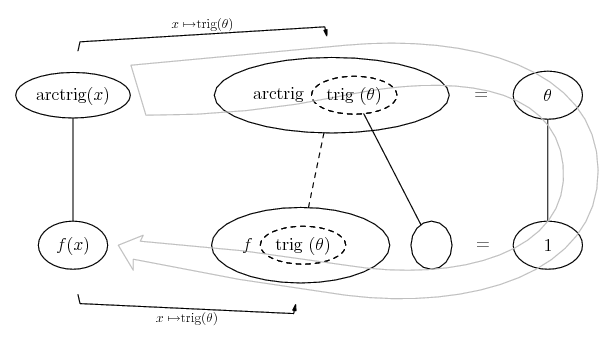# Thread: Help with Gradient Of function problem

1. ## Help with Gradient Of function problem

Suppose (x,y) is a point in the first quadrant (x,y>0). If delta is the angle of (x,y) in polar representation, determine the length and direction of gradient of delta(x,y)?
can someone show me how to approach this type of question?
Thanks for the help!

2. ## Re: Help with Gradient Of function problemOriginally Posted by Ricebunnythe angle of (x,y) in polar representation
... equals arctan (y/x).

arctan(y/x) - Wolfram|Alpha

Notice the partial derivative wrt x. Click on 'show steps'. Just in case a picture helps...... where (key in spoiler) ...

Spoiler:... is the chain rule. Straight continuous lines differentiate downwards (integrate up) with respect to the main variable (in this case x), and the straight dashed line similarly but with respect to the dashed balloon expression (the inner function of the composite which is subject to the chain rule).

The general drift is...... equals a vector composed of both partial derivatives:

Gradient - Wikipedia, the free encyclopedia

gradient of arctan(y/x) - Wolfram|Alpha

determine the length and direction
.. as for any vector: the length or magnitude using pythagoras and the direction relative to the x-axis using arctan as we did above for delta.

For length, simplify $\displaystyle \sqrt{{(\tfrac{-y}{x^2 + y^2})}^2 + {(\tfrac{x}{x^2 + y^2})}^2}$

For direction, arctan of a simplification of the 'y' component over the 'x'.

_________________________________________

Don't integrate - balloontegrate!

Balloon Calculus; standard integrals, derivatives and methods

Balloon Calculus Drawing with LaTeX and Asymptote!

3. ## Re: Help with Gradient Of function problem

Thank you so much for the help!
Now I understand much better.

#### Search Tags

function, gradient, problem